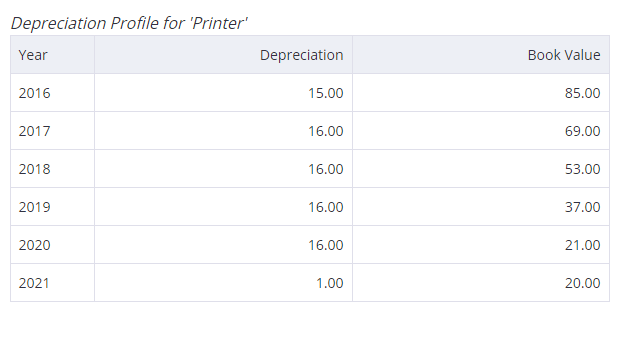Debitoor's accounting dictionary
Depreciation methods

# Depreciation methods – What are depreciation methods?

Depreciation methods are different ways of calculating how much value goods lose throughout their expected lifetime.

Debitoor calculates depreciation automatically, making it even easier to keep track of your business’s assets. Try Debitoor for free with our seven-day trial.

Although assets play a key role in helping businesses provide goods or services, they become less valuable and less productive throughout their useful life. It’s therefore important that the cost of an asset is divided throughout its expected lifetime in a way that considers it’s declining value.

This is known as depreciation, and there are several different depreciation methods, which allow businesses to determine the projected loss of value of certain assets over time or based on actual physical usage. This allows for an effective allocation of costs throughout the useful life of the asset in the correct period.

## The four main depreciation methods

There are four main depreciation methods. Three of these methods are based on time, while one is based on actual usage.

### Straight-line depreciation method

Straight-line depreciation is the most simple and commonly used depreciation method.

You can calculate straight-line depreciation by subtracting the asset’s salvage value from the original purchase price and then dividing it by the total number of years it is expected to be useful for the company. The straight-line depreciation method results in equal depreciation expenses spread evenly over the course of the asset’s useful life.

### Reducing balance depreciation method

Reducing balance depreciation changes the amount of depreciation charged over time. It is considered an ‘accelerated’ depreciation method.

Reducing balance depreciation method is most useful for assets that typically lose the most value in earlier years, but then experience a slowing of depreciation later on. Computer equipment is a good example of an asset that would benefit from this method.

### Sum-of-the-year’s-digits depreciation method

Sum-of-the-year’s-digits depreciation is also an accelerated depreciation method, allocating higher amounts of depreciation to the earlier years of an asset’s useful life and lower amounts towards the end.

The sum-of-the-year’s-digits depreciation method is determined by adding up the total number of years of the asset’s expected useful life and then figuring out the year in which it currently falls on that scale.

### Units of production depreciation method

Whereas the other three methods of depreciation use time to estimate how much value an asset has lost, the units of production depreciation method takes into account the amount of activity the asset actually experiences. For example, it allows for a higher depreciation rate during periods of high usage, and a lower rate for periods of low usage or idleness.

The units of production depreciation method is useful for assets that are greatly affected by physical use or output.

## Which depreciation method should I use?

It can be difficult to know which depreciation method you should use, there are pros and cons of using each method of depreciation.

When choosing between the four different methods of depreciation, you should consider the nature of your assets. For example, you should think about whether they become less productive and useful at an even rate or whether they suddenly drop in value.

Similarly, you should consider whether you will use your asset at regular, steady intervals or whether it might be idle for long periods of time.

Finally, it’s important to consider whether it’s necessary to use complex methods of depreciation or whether a more simple method will be enough. Whereas larger organisations might want to use the most accurate depreciation method, for most small businesses and freelancers, straight-line depreciation is simple enough to calculate and sufficient for considering how an asset declines in value.

## Depreciation methods in Debitoor

With Debitoor invoicing software it’s easy to keep track of your business assets. If you indicate that an expense is actually an asset, you’ll be prompted to enter it’s expected useful life and residual value.

Our software then automatically calculates depreciation expenses using the straight-line method, giving you a ‘Depreciation Profile’.